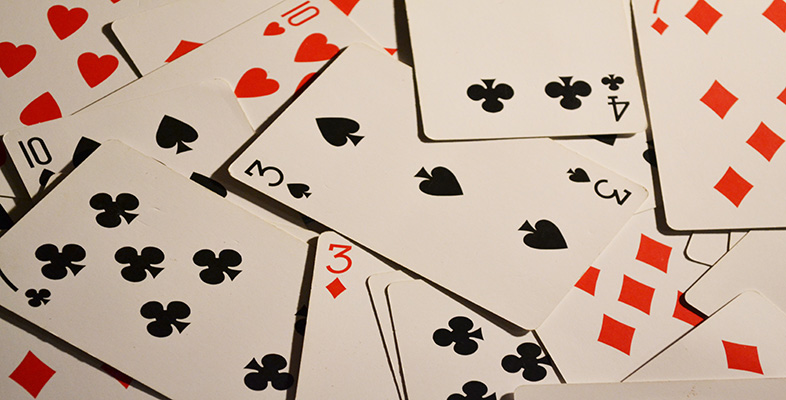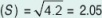Investment risk

Start this free course now. Just create an account and sign in. Enrol and complete the course for a free statement of participation or digital badge if available.

Free course

# 2.2 Calculating returns

Our first question was: what is the mean expected total return for next year? We calculate this by taking each of the possible returns and weighting it by its relative probability. As our table is so simple and symmetrical, it is not difficult to see that the weighted mean return is 7% per annum.

Our second question was: what is the degree of risk or uncertainty in this mean figure? In other words, how widely dispersed are the possible outcomes around the mean expected outcome? The most commonly used statistical measure of dispersion is the standard deviation. We need to be aware of some limitations in its use, but first here is how the calculation would look in the case of returns on Company X's shares. We shall use the notation E (R) to denote the mean expected return, which is 7% per annum in this case.

## Table 2 Calculation of standard deviation of actual total returns on a one-year investment in shares of Company X in each of the last 50 years

Annual return RIDispersion E﻿(﻿R﻿) − R﻿iSquare of dispersion [﻿E﻿(﻿R﻿) − R﻿I﻿]﻿2Probability PIP﻿i﻿[﻿E﻿(﻿R﻿) − R﻿i﻿]﻿2
4+390.21.8
6+110.30.3
8−110.30.3
10−390.21.8

Sum of the squares of the probability-weighted dispersions (= variance) V = 4.2

Square root of variance (﻿V﻿)﻿ = standard deviationThe standard deviation is normally expressed in the same units as the expected return (in this case, per cent per annum) and is intuitively easier to understand than the variance, especially with a normal distribution where the possible outcomes are symmetrically dispersed around the mean.

The particular usefulness of the standard deviation of normally distributed data is the way it divides up the data so that:

• 68.3% of the data points lie within one standard deviation on either side of the mean;

• 95.4% of the data points lie within two standard deviations on either side of the mean.

In the case of an investment in shares of Company X, the calculation in Table 2 tells us that there is:

• a 68.3% probability that the return will lie between 4.95% (i.e. 7 – 2.05) and 9.05% (i.e. 7 + 2.05), and

• a 95.4% probability that it will lie between 2.9% (i.e. 7 – 2 x 2.05) and 11.1% (i.e. 7 + 2 x 2.05).

The principal limitation on the use of standard deviation as a measure of dispersion in investment returns is that in the real world actual returns are not as neatly or symmetrically dispersed as they were in our Company X example. However, the shape of many long-run statistical series for investment returns is in fact quite close to the familiar bell-curve of the normal distribution. So for most practical purposes the standard deviation is as useful a measure as we are likely to find.

Try these techniques for yourself in the following self-assessment.

## Self-assessment question 1

1. Calculate the mean expected annual return on shares in The Lead Balloon Co. Ltd from the following historical data.

Total return (% per annum)Frequency of occurrenceProbabilityProbability-weighted return
−7%4
3%15
10%18
15%24
21%19
26%12
29%8
Total/Mean100

2. What assumptions did you make in your calculation?

3. Now take your results from the first part of the self-assessment and use the following table to calculate the standard deviation of the same set of returns.

Annual return RIDispersion E﻿(﻿R﻿) − R﻿iSquare of dispersion [﻿E﻿(﻿R﻿) − R﻿I﻿]﻿2Probability PIP﻿i﻿[﻿E﻿(﻿R﻿) − R﻿i﻿]﻿2
−7%
3%
10%
15%
21%
26%
29%

Sum of the squares of the probability-weighted dispersions (= variance) =

Square root of variance (V) = standard deviation (S) =

4. Does the table show a normal distribution?

5. What do you think is the probability that the actual rate of return in any year might lie between 6% and 24%?

1.

Total return (% per annum)Frequency of occurrenceProbabilityProbability-weighted return (% per annum)
−740.04−0.28
3150.150.45
10180.181.80
15240.243.60
21190.193.99
26120.123.12
2980.082.32
Total/Mean10015.00%

2. The main assumption that you made was that the future can be extrapolated from the past. You may well take the view that demand for this particular company's product is unlikely to fluctuate substantially over time!

3.

Annual return RIDispersion E﻿(﻿R﻿) − R﻿iSquare of dispersion [﻿E﻿(﻿R﻿) − R﻿I﻿]﻿2Probability PIP﻿i﻿[﻿E﻿(﻿R﻿) − R﻿i﻿]﻿2
−7%224840.0419.36
3%121440.1521.60
10%5250.184.50
15%000.240
21%−6360.196.84
26%−111210.1214.52
29%−141960.0815.68
82.50

Sum of the squares of the probability-weighted dispersions (= variance)

Square root of variance (V) = standard deviation (S) = 82.50 = 9.083

4. The table shows a pattern quite close to a normal distribution. The mean and mode do coincide at 15.00%. There is a slight asymmetry in the shape of the ‘shoulders’ of the bell. The ‘tails’ at opposite ends of the distribution differ from each other slightly, but not so much as to skew the data significantly in one direction or the other. Overall, we can say that standard deviation is still a useful measure of dispersion for a table of this type.

5. The standard deviation of 9.083% means that there is a 68.3% probability that the actual return will lie between (15.00 – 9.083)% and (15 + 9.083)%, i.e. between approximately 5.9% and 24.1%. The probability of an outcome between 6.00% and 24.00% is therefore slightly less than this – say, about two-thirds.

B821_1